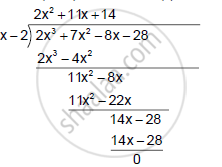Share

Using Remainder Theorem, Factorise: 2x3 + 7x2 − 8x – 28 Completely - ICSE Class 10 - Mathematics

ConceptFactorising a Polynomial Completely After Obtaining One Factor by Factor Theorem

Question

Using remainder Theorem, factorise:

2x3 + 7x2 − 8x – 28 Completely

Solution

Let f (x) = 2x3 + 7x2 − 8x − 28
For x = 2,
f(x) = f(2) = 2(2)3 + 7(2)2 − 8(2) − 28 = 16 + 28 − 16 − 28 = 0
Hence, (x − 2) is a factor of f(x).∴ 2x^3 +  7x^2 - 8x - 28 =( x - 2)( 2x^2 + 11x + 14)
= (x- 2) ( 2x^2 + 4x + 7x  + 14)
= (x - 2) [ 2x (x + 2)+ 7( x+ 2)]
= (x -2) (x +2)( 2x + 7)

Is there an error in this question or solution?

APPEARS IN

Solution Using Remainder Theorem, Factorise: 2x3 + 7x2 − 8x – 28 Completely Concept: Factorising a Polynomial Completely After Obtaining One Factor by Factor Theorem.
S